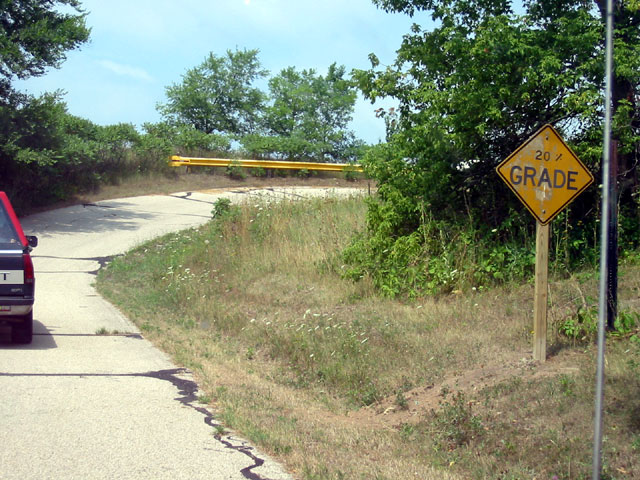chapter 7 right triangles and trigonometry

Section 7.2 The Pythagorean Theorem and Its ConversePythagorean TheoremIn a right triangle, the sum of the squares of the legs equals the square of the hypotenuse.The legs are represented as a and b, the hypotenuse is represented as c.There are two shortcuts that can usually be used that involve the Pythagorean Theorem:

To find the Hypotenuse (c):

1. square the legs

2. Add them together

3. Take the square root of the sum

To find a Leg (a or b):

1. square the hypotenuse and the known leg

2. Subtract them

3. Take the square root of the difference

Example:Example:Converse of the Pythagorean Theorem: If the sum of the squares of two sides equals the square of the third side, then the triangle is right.

So if, then the triangle is right.

Example:Is this a right triangle?

Pythagorean Triples:  three whole numbers that represent the sides of a right triangle.

Some common examples of Pythagorean triples:

3-4-5, 6-8-10, 9-12-15, 30-40-50, 5-12-13, 8-15-17, 7-24-25, 20-21-29 and there are many more examples

Can these three lengths be the sides of a right triangle?

Then state whether they form a Pythagorean triple.

10, 15, 20                    9, 40, 41            3/8,  4/8,  5/8Area of Right Triangles

Area = Base (one leg) * height (other leg) / 2

Section 7.3 Special Right Triangles

45-45-90 Triangle:ORLegs are congruent30-60-90 Triangle:Examples:Other Examples:

The perimeter of an equilateral triangle is 60 inches.  Find the length of an altitude.

The diagonal of a square is 14.  Find the length of a side and then find the perimeter of the square.

The altitude of an equilateral triangle is 10.  Find the length of a side and then find the perimeter of the equilateral triangle.

Section 7.4 Trigonometry

Trig Ratios:  SohCahToa

Sine (sin):  opposite leg / hypotenuse

Cosine (cos):  adjacent leg / hypotenuse

Tangent (tan):  opposite leg / adjacent leg

Example:10*sin38 = x              10 * cos38 = y

Example:y = 10 / cos42                10 * tan42 = x

Example:y = 8 / sin64                                        x = 8 / tan64

Section 7.4 (part 2)

Trig can be used to find the angle measures of a right triangle as well.

Use the inverse of the sine or cosine or tangent to calculate the measure of an angle.

Solve the Right Triangle (find all unknown measures)Section 7.5 Angles of Elevation and Depression

Angle of ElevationAngle between line of sight and horizontal when looking upward.Angle of DepressionAngle between line of sight and horizontal when looking downward.Example 1:  The angle of elevation from point A to the top of a cliff is 34 degrees.  If point A is 1000 feet from the base of the cliff, how high is the cliff?

Example 2:  The angle of depression from the top of an 80 foot building to point B on the ground is 42 degrees.  How far from the base of the building is point B?

Example 3:  The tailgate of a truck is 3.5 feet above the ground.  A loading ramp is attached to the tailgate with an incline of 10 degrees.  Find the length of the ramp.

Example 4:  A sledding hill is 300 yards long with a vertical drop of 27.6 yards.  Find the angle of depression of the hill.

Percent Grade is calculated by dividing the rise (vertical elevation) by the run (horizontal distance)

Example 5:  What is the angle of elevation of these hills?Steepest street in the world 35% in New ZealandGreen slopes are usually between 6% and 25% grade

Blue slopes are between 25% and 40%

Black slopes are greater than 40%

What is the angle of depression range for each slope?

Ian is standing on the ground 60 feet from a cliff looking up to the top with an angle of elevation of 500. His eye level is 6 feet above ground.

How tall is the cliff?

Now Ian is standing on top of a 40 foot building looking down at Teren with an angle of depression of 120. His eye level is 6 feet above the top of the building.

How far is it from the car to his eyes?